« One-way slabs Deflection »

Static analysis

One-way slabs are those supported on two opposite edges, such as slab s1 in figure of §4.1.

If a one-way slab is supported on more than two edges and its aspect ratio, i.e. the ratio of the larger to the smaller theoretical span, is greater than 2.0 (such as slab s3 in the same figure), it is considered as one-way slab in the principal direction while taking into account the secondary stresses in rest edges.

Continuous one-way slabs are analysed considering a frame of continuous bars of rectangular shape cross-section, having width equal to 1.00 m and height equal to the thickness of the slab. The strip loads comprise self-weight, dead and live loads.

Analysis is performed:

a) approximately, by applying all design loads p=1.35g+1.50q (when live load is relatively small)

b) accurately, by taking into account unfavourable loadings.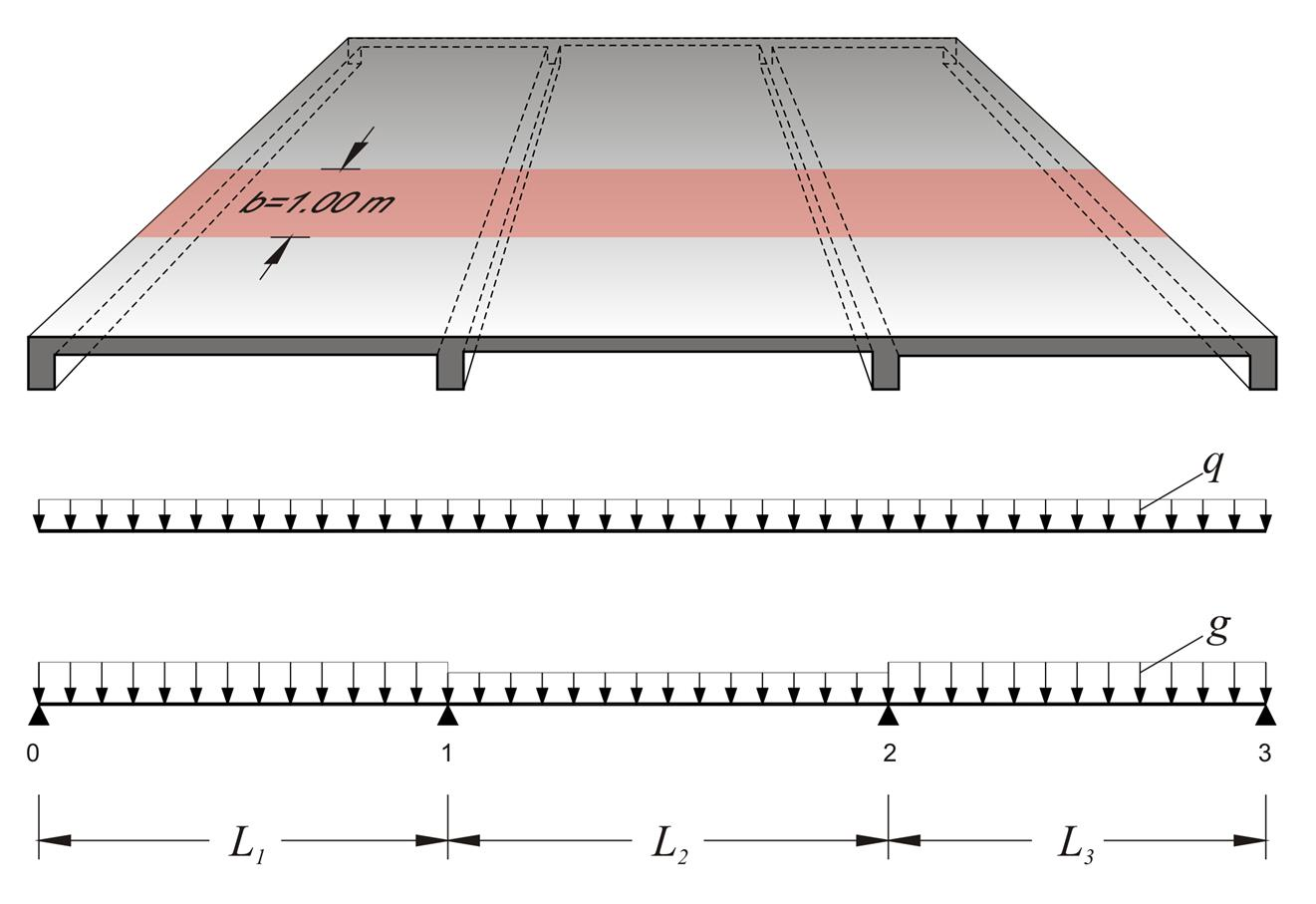Figure 4.5.1-1: Three-span continuous slab
Figure 4.5.1-1: Three-span continuous slab

Example:

The three slabs (previous figure) have L1=4.50 m, h1=180 mm, g1=10.0 kN/m2, q1=2.0 kN/m2, L2=4.00 m, h2=140 mm, g2=5.0 kN/m2, q2=2.0 kN/m2, L3=4.00 m, h3=140 mm, g3=5.0 kN/m2, q3=2.0 kN/m2, where loads g include self-weight. Perform static analysis considering global loading in ultimate limit state.

Design load in each slab is equal to pig·giq·qi=1.35·gi+1.50·qi, thus on 1.00 m wide strip, it is:

p1=1.35x10.0+1.50x2.0=16.5 kN/m

p2=p3=1.35x5.0+1.50x2.0=9.75 kN/m

The three-span continuous slab will be solved through Cross method.

Fundamental design span moments (table b3)

M10=-p1·L12/8=-16.5x4.502/8=-41.8 kNm

M12=M21=-p2·L22/12=-9.75x4.002/12=-13.0 kNm

M23=-p3·L32/8=-9.75x4.002/8=-19.5 kNm

Moments of inertia I

I01=Ic=1.0x0.183/12=4.86x10-4 m4

I12=I23=1.0x0.143/12=2.29x10-4 m4=0.47Ic

Stiffness factors k, distribution indices υ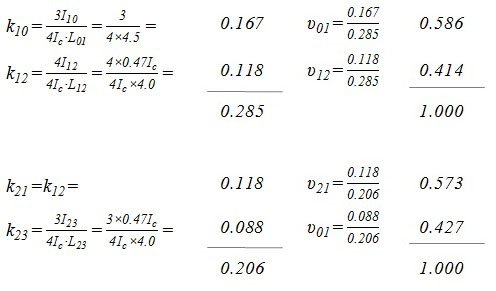1 2 0.586 0.414 0.573 0.427 +41.8 -[+41.8-13.0]x0.586→ - 16.9 -[+3.6]x0.586→ - 2.1 -[+0.3]x0.586→ - 0.2 -13.0 -11.9 + 3.6 - 1.5 + 0.3 - 0.1 >0.50 0.50← >0.50 0.50← +13.0 - 6.0 + 7.2 - 0.8 + 0.5 -19.5 + 5.3 ← 0.427x[-(+13.0-19.5-6.0)] + 0.3 ← 0.427x[-(-0.8)] +22.6 -22.6 +13.9 -13.9 M1=-22.6 kNm M2=-13.9 kNm

V01=16.5x4.50/2-22.6/4.50=32.1 kN

V10=-16.5x4.50/2-22.6/4.50=-42.1 kN

V12=9.75x4.00/2+(-13.9+22.6)/4.00=21.7 kN

V21=-9.75x4.00/2+(-13.9+22.6)/4.00=-17.3 kN

V23=9.75x4.00/2+13.9/4.00=23.0 kN

V32=-9.75x4.00/2+13.9/4.00=-16.0 kN

maxM01=32.12/(2x16.5)=31.2 kNm

maxM12=21.72/(2x9.75)-22.6=1.5 kNm

maxM23=16.02/(2x9.75)=13.1 kNm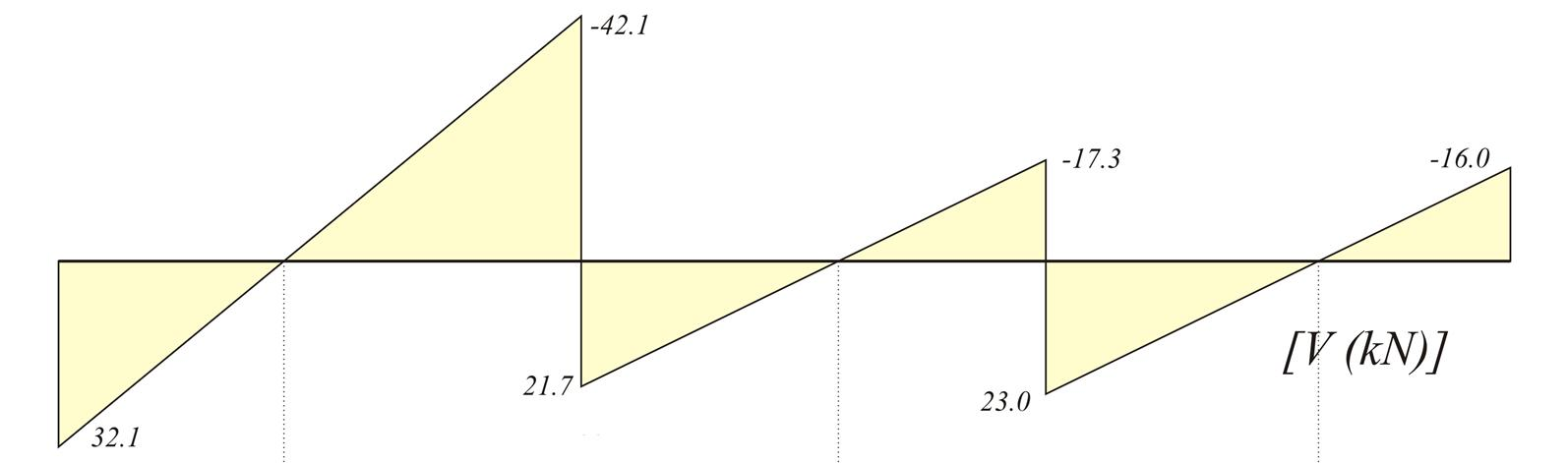Figure 4.5.1-2: Shear force diagram
Figure 4.5.1-2: Shear force diagram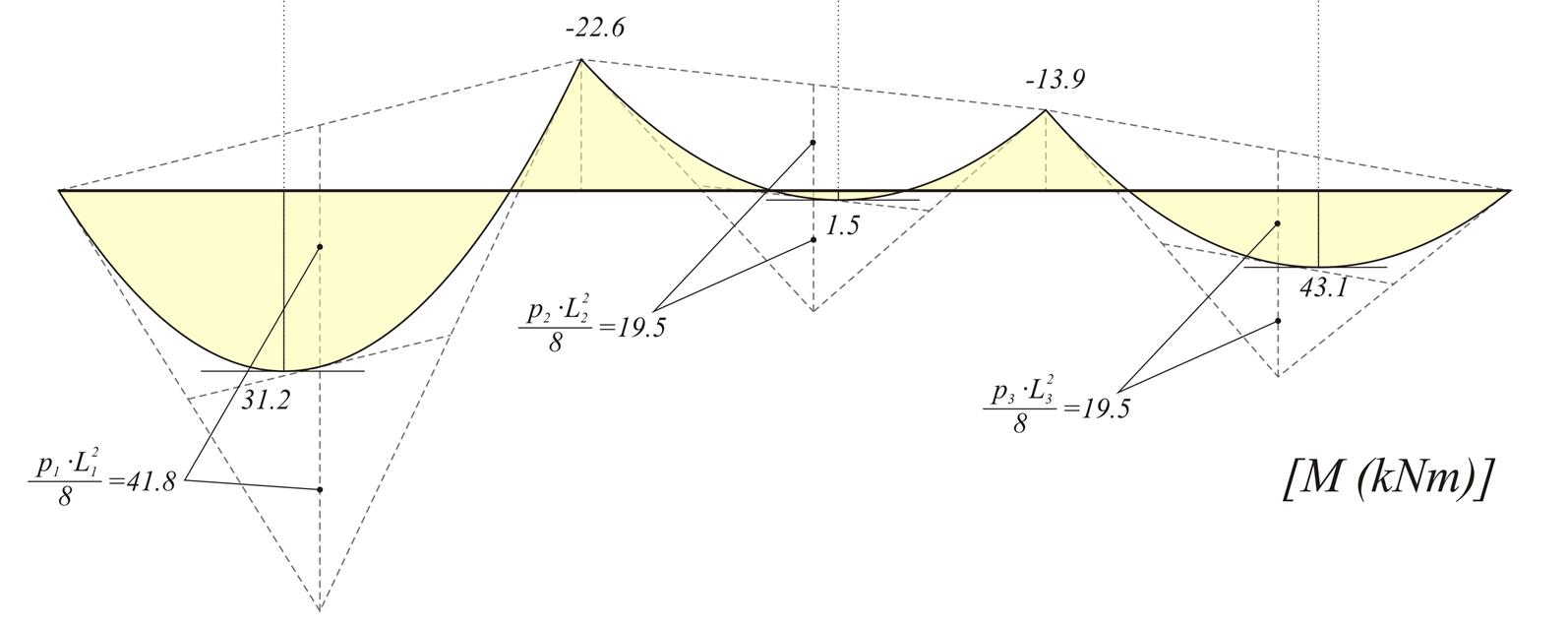Figure 4.5.1-3: Bending moment diagram
Figure 4.5.1-3: Bending moment diagram

Note:

Project <B_451> using Standard Solver gives:

V01=32.1 kN      Μ1=-22.7 kNm

V10=-42.2 kN     M2=-13.8 kNm

V12=21.7 kN      maxM01=31.2 kNm

V21=-17.3 kN     maxM12=1.6 kNm

V23=23.0 kN      maxM23=13.2 kNm

V32=-16.1 kN

Project <B_451> using pi-FES gives:

V01=31.7 kN      Μ1=-22.3 kNm

V10=-41.8 kN     M2=-13.4 kNm

V12=21.5 kN      maxM01=31.2 kNm

V21=-17.1 kN     maxM12=1.5 kNm

V23=22.7 kN      maxM23=13.2 kNm

V32=-15.8 kN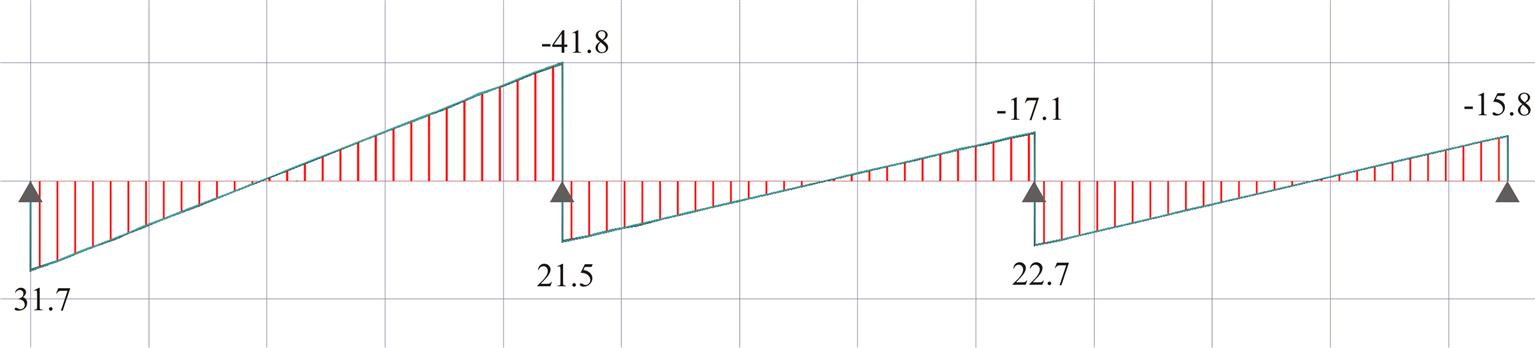Figure 4.5.1-4: Shear force diagram (from pi-FES with active module\SLABS)
Figure 4.5.1-4: Shear force diagram (from pi-FES with active module\SLABS)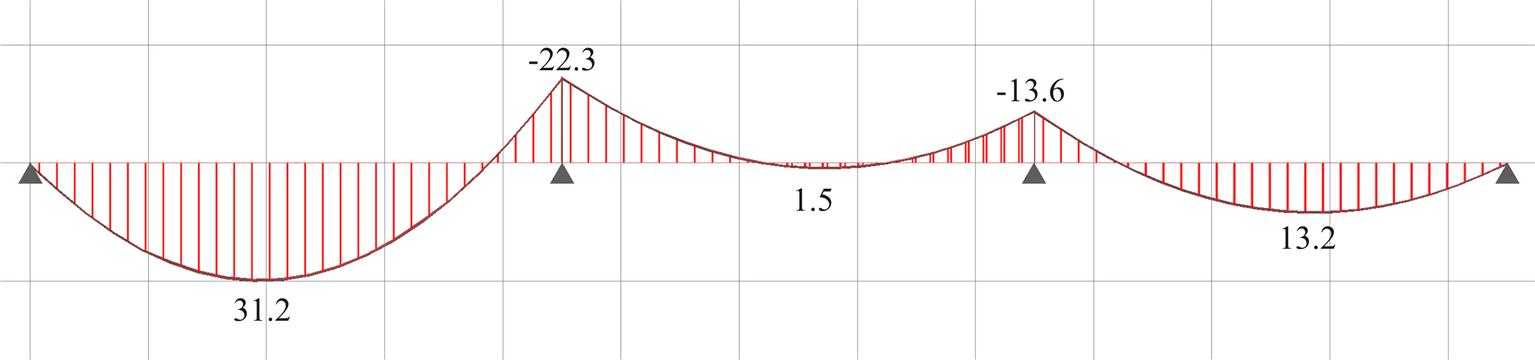Figure 4.5.1-5: Bending moment diagram (from pi-FES with active module\SLABS)
Figure 4.5.1-5: Bending moment diagram (from pi-FES with active module\SLABS)

« One-way slabs Deflection »